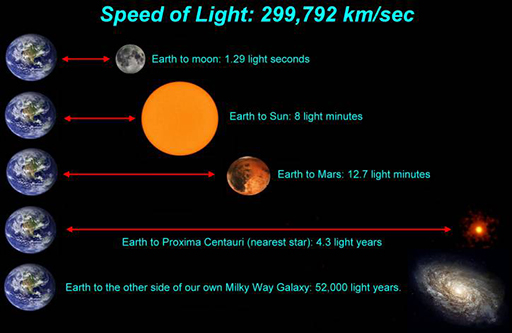Science, Maths & Technology

### Become an OU studentAn introduction to exoplanets

Start this free course now. Just create an account and sign in. Enrol and complete the course for a free statement of participation or digital badge if available.

# 4  The light year

Because space is so big, we need to use another measurement of distance to think about the distances between stars. To recap, so far, we’ve measured:

• planet sizes in units of RE and RJ
• star sizes in units RSun
• distances within planetary systems in units of AU, which is the distance between the Earth and the Sun.

To measure distances between stars, we use a unit called the ‘light year’.

The light year is simply the distance light can travel in a year. To us, it looks as though light travels instantaneously, but actually it’s just extremely fast. Light travels at a speed of nearly 300 000 kilometres every second – fast enough to take it over seven times around the Earth in just one second!

This is the fastest known thing in the Universe. Based on existing scientific theory, it’s actually impossible for anything to travel faster than the speed of light. Even so, it still takes light eight minutes to travel from the Sun to us, and the light we see from our nearest neighbouring star, Proxima Centauri, left over four years ago (Figure 4).

There are 31.5 million seconds in a year, so light can travel a distance of 300 000 × 31.5 million km in a year. A light year works out as approximately 9.5 × 1012 km (remember, 1012 is 1 with 12 zeros after it – 1000 000 000 000, or 1 million million, or 1000 billion, or 1 trillion, though scientists don’t tend to use the word ‘trillion’).Figure 4  Light-speed distances

## Activity 3  Light-speed distances

If each of the distances in Figure 4 was drawn to the same scale, with, say, 1 cm representing the distance from Earth to the Moon, then the distance across the Milky Way Galaxy should be represented by a line 13 million kilometres long!

Can you work out and explain how we get 13 million km from the information in Figure 4?

First, we need to convert 52 000 light years into light seconds so we can compare it with the Earth–Moon distance in the same units, light seconds. To do this we must multiply by the number of days in a year and the number of seconds in a day. So, the distance across our Galaxy in light seconds is:

52 000 light years × (365.25 × 24 × 60 × 60) seconds per year

= 1640 995 200 000 light seconds

= 1.6 × 1012 light seconds.

Since the distance to the Moon, 1.29 light seconds, corresponds to 1 cm, the scale would be 1.29 light seconds per cm. We need to divide the distance across the Galaxy by the 1.29 light seconds per cm to work out the width of the figure in cm if drawn to scale:

1.6 × 1012 light seconds ÷ 1.29 light seconds per cm

= 1.3 × 1012 cm.

This is a very big number, so it makes sense to express the answer in km rather than cm. There are 100 cm in 1 m and 1000 m in 1 km, so our answer is:

1.3 × 1012 cm ÷ 100 = 1.3 × 1010 m

1.3 × 1010 m ÷ 1000 = 1.3 × 107 km.

Since 1 million is 1 × 106, 1.3 × 107 is the same as 1.3 × 10 × 1 million. So, our answer 1.3 × 107 km is more elegantly expressed as 13 million km.

That is, if Figure 4 was drawn to scale in which the distance to the Moon is 1 cm, then we would need a piece of paper 13 million km long!

As we said, space is really, really big! This is why some pictures in astronomy are not drawn to scale.

What is the true distance from Earth to the other side of the Milky Way in kilometres?

A light year is a distance of 9.5 × 1012 km, so 52 000 light years is a distance of

9.5 × 1012 × 52 000 = 5 × 1017 km.

That’s 5 hundred thousand million million – phew!

In fact, recent studies of the outermost stars in the Milky Way have suggested that it may be even bigger than that.

And that’s just the distance across our own Milky Way Galaxy! There are many billions of other galaxies out there.

## Activity 4  How long would it take the light to get here?

In Figure 4, you’ve been shown how long it would take light to reach the Earth from different objects. Based on those numbers, see if you can work out how long it would take light to come from elsewhere.

1. If Mercury is about half the distance from the Earth to the Sun, how long does it take light from the Sun to get to Mercury?

4 minutes

1. The International Space Station (ISS) is about 1000 times closer to the Earth than the Moon is. Approximately how long does it take light to reach us from the ISS?